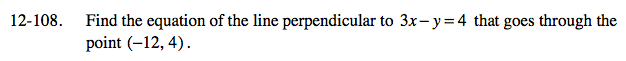### Home > AC > Chapter 12 > Lesson 12.4.4 > Problem12-108

12-108.

Find the equation of the line perpendicular to 3x − y = 4 that goes through the point (−12, 4). Homework Help ✎Solve the equation for y.

The slope of a perpendicular line is the opposite reciprocal of the original line.

Use the slope of the line and the point to solve for b.